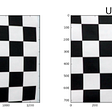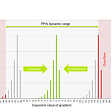# Battery capacity estimation using Machine Learning : Part-2

This is a two part blog series on understanding and predicting battery capacity using machine learning. In the part-1 of battery capacity estimation with machine learning, EDA and data preprocessing is discussed. In this part, feature engineering, modeling and results are discussed.

• Feature Extraction
• Modeling
• Results
• Future work
• References

# 5. Feature engineering

## 5.1 Charge cycle:

For charge cycles, the following features are analyzed and extracted.

• Duration in Constant current mode
• Decay of current
• Peak temperature over mean

CC mode duration

This indicates the duration of charging in which the cell stayed in constant current while charging. Since charging was carried out in a constant current mode at 1.5A, Time column for indices corresponding to 1.5 are obtained and converted into minutes.

Decay of current

In charging mode, once mode shifts to CV mode, current steadily decays and eventually enters trickle mode. During this process, the current decay can be observed if it has any relation with capacity by fitting a polynomial curve and observing coefficients.

The above plots are coefficients of a curve fitted to the decaying current which can be interpreted as a curve. The order of the curve (np.polyfit) is obtained by manually plotting and chosing the best order in which the curve is not fitting overly to the cycle data. These are the variation of coefficients of fitted curves on charge cycle over increasing order of cycles. These coefficients capture decay rate duration shifts that happen when the cell/battery loses capacity. The coefficients are very low in magnitude

Peak temperature over median

Difference between median temperature and peak temperature is calculated

`peak_temp_med = [max(d['data']['Temperature_measured']) - np.median(d['data']['Temperature_measured']) for d in charge_data]`

The difference is increasing with a significant trend to it, but it is irregular in nature as well. This curve can be processed through SG-filter to obtain smoothed values which can be used as a feature

## 5.2 Discharge Cycle:

For discharge cycles, the following features are analyzed and extracted

• CC mode duration
• Recovery voltage magnitude
• Decay rate of voltage
• growth parameters of temperature in CC mode

CC mode duration

Duration of cycle which stayed in constant current mode while discharging.

The above plot is Change of CC mode duration in discharge mode over multiple cycles. The decrease in trend is similar to the decrease in trend of the target variable.

Recovery voltage magnitude

The voltage that the battery gains, once the discharge is completed is the voltage recovered. The magnitude of the recovery voltage is different as the cycle reaches EOL.

The recovery voltage seems to increase over time clearly with some irregularity in the initial measurements

Decay rate of voltage

In the discharge cycle, the voltage decreases over time. A polynomial curve is fitted and coefficients are extracted.

Coefficients of fitted curves for voltage decay are reducing consistently on how there is a decrease in voltage duration as cycles increase.

Rate of temperature in CC mode:

During discharge, as long as the current stays constant, the temperature steadily rises. The variation of rise of temperature can be observed by fitting a polynomial curve and plotting coefficients.

Three of the four coefficients are almost linearly increasing/decreasing. 4th coefficient, which is the main component, is not consistent in any direction.

## 5.3 Sequential Spectrum Analysis

In previous methods, feature information is extracted by looking at only one cycle information. As the battery capacity degrades, there are noticeable differences in charge — discharge profiles. This difference between cycles is not noticeable when looked at two consecutive cycles, but are observable when looked a few cycles away from the current cycle. These cycles also contain a lot of noise while logging which makes point to point comparison difficult. The consecutive method uses SSA to remove periodic noise, fluctuations etc from the main cycle data which are then compared with previous cycles. For each current cycle, past 10 cycles data can be used to obtain relative change in profiles which captures/correlates to reduction in capacity over time.First two components are as follows.

Similarity based analysis

A window of 10 previous cycles is averaged and then compared with current cycle data to obtain an estimate of similarity. Dot product used to obtain similarity between the cycles. To compute the dot product, the two inputs should be of the same size, So from each cycle, percentiles are extracted. The similarity based variation is shown below.

The similarity with SSA is processed for charge voltage, discharge voltage, charge current and discharge current.

## 5.4 Fourier transform

Similar to SSA, Fourier transform is applied on the ten cycles, frequencies are extracted. The components of the frequencies are separated and categorized based on cycles.

# 6. Models

The dataset after feature engineering contains a very low number of points. Train data contains 68 rows and test data contains relatively higher 99 rows. The training can be done with 68 datapoints but there is a possibility of overfitting. Hence the train data is upsampled using linear interpolation between cycles. This means that fake cycles data is created based on actual cycle data. However, there is a need to know if the data interpolation made any difference in performance of the models. So initially, the models are fitted on the sparsely available data to obtain a ‘baseline’ like metrics.

## No interpolation

SGD regressor

SVM regressor

RANSAC regressor

Decision Tree

Random forest

GBDT

Models with best parameters

Models with best hyperparameters used above are all gathered to understand relative performance.

• It can be seen that Random forest seems to be doing well when compared to all other models
• Decision tree seems to be doing better than RF but it is overfitting which resulted in higher difference in train and test metrics when compared to RF model

## Interpolation

Method of interpolation:

• Sort the training data based on cycle column
• expand_len : number of observations to which the existing dataset is to be interpolated
• dummy_row_len = expand_len/existing_dataset_rows, gives how many new points are needed to be generated between two rows of existing dataframe rows
• Create new dataframe with rows equal to expand_len and columns equal to existing dataframe and value of np.nan
• for every dummy_row_len, add a row from existing dataframe into new df
• for each column, use pandas.interpolate function to obtain nan values
`DF_upsamp = interpolate_data(DF_train, 1020)`

After interpolation, the train data size is increased to 1020 datapoints

SGD regressor

SVM regressor

RANSAC regressor

Decision tree regressor

Random forest regressor

GBDT

LGBM

Relative comparison of models with best hyperparameters.

Random forest and LGBM are close best performers with LGBM slightly in lead. Linear models are not great performers in this case and GBDT performs worse than linear regression. LGBM is added to data to verify the performance of standard boosted models other than sklearn GBDT.

Custom stacking model

A custom stacking model is implemented which is a bootstrapped ensemble of multiple models and a meta model. The process of the custom model is described below.

• The entire dataset is separated into train and test set (80–20)
• The train set(80%) is splitted into two sets D1 and D2 (50–50)
• On the dataset D1, Sampling with replacement is done to create sub-datasets d1, d2, d3..dk(k samples)
• With the k samples, k models are trained with each sample set in k. This creates k base models which are trained on slightly different k datasets.
• Using the K models, predict K predictions on the dataset D2. The K predictions for each point in D2 is used to train a meta model.
• The test set (20%) which is left out in the beginning is used as an evaluation set to understand the performance of the custom model.

In order to implement this process, a custom class is implemented which includes k datasets creation, base model training and meta model training. The custom model is implemented in three different ways.

1. Fixed base model hyperparameters : The base models are with hyperparameters which performed best in previous training.
2. Randomized hyperparameters: The base models are top 3 or 4 performing models in previous training and are picked randomly.
3. Flexible base model: The base models can be explicitly mentioned during the custom model initiation. If no models are mentioned, it falls back to fixed base model type custom model. Below is the code for the flexible base model. This is chosen as the final custom model as it performed better than the above two types of custom models after tuning with various combinations of base models.

Arguments for custom model :

• sample_k- No of base models
• sample_size- No of data samples per base model
• base_models- List of base models to choose. In np.nan, models are chosen randomly which adds more randomness to the custom model

The custom model is trained and the performance metrics MAE and MAPE are tracked.

`mae = []mape = []for _ in tqdm(range(500)):  model = Custom_model(16, 256, base_models = ['RF','LGBM'])  model.fit(X_train_1, X_train_2, y_train_1, y_train_2)  y_test_pred = model.predict(X_test)  mae.append(mean_absolute_error(y_test,y_test_pred))  mape.append(mean_absolute_percentage_error(y_test,y_test_pred))`

The custom model has MAE of 0.00608 and MAPE of 0.35%. This data when included along with the previous models gives a better context of the performance.

# 7. Results

The best set of models from each category i.e no-interpolation, interpolation and interpolation + custom models.

From no interpolation to interpolation of data, there is a significant improvement in performance. The custom model also performed better than Random forest with no interpolation but was slightly behind the performance of LGBM.

# 8. Deployment

The model is deployed locally using streamlit which takes cycle number as input and generates capacity as output.

# 9. Future work

• A dataset with features having varying load would be more useful as applications tends to have varying load.
• Dependency on previous cycles can be removed.
• Application specific data will be more insightful in understanding load variation and capacity variation.

# 10. References

For any doubts, or queries, the readers may comment in the blog post itself, or connect with me on LinkedIn.

You can find the code in github

--

--

--

## More from R.A.M

learning and improving

Love podcasts or audiobooks? Learn on the go with our new app.

## Torch — Logistic Regression on Iris dataset## Cracking the Black Box of Healthcare## How to compute gradients in Tensorflow and Pytorch## Advanced Lane Lines — Challenge Videos Try## Torch: Spoken digits recognition from features to model.## AI, Sustainability Tweets: Sentiment Analysis Using Pre-trained Models## Using Jax to streamline machine learning optimization## Simplify mixed precision training with MXNet-AMP## R.A.M

learning and improving

## Battery capacity estimation using Machine Learning : Part-1## My Background Going into the Flatiron School’s Data Science Program — Blog #1